Which point is located in Quadrant IV?

A coordinate plane.
A(-8, 6)
B(-8, -6)
C(8, -6)
D(8, 6)

Result

x = (Correct answer is: C)### Step-by-step explanation: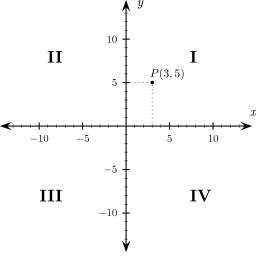Did you find an error or inaccuracy? Feel free to write us. Thank you!Tips to related online calculators
Line slope calculator is helpful for basic calculations in analytic geometry. The coordinates of two points in the plane calculate slope, normal and parametric line equation(s), slope, directional angle, direction vector, the length of the segment, intersections of the coordinate axes, etc.

## Related math problems and questions: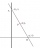On a graph, Point Y is located at (4, -2). Point Z is located 5 units to the left of Point Y. In which quadrant is Point Z located?
• Midpoint 4If the midpoint of a segment is (6,3) and the other endpoint is (8,-4), what is the coordinate of the other end?
• Coordinates of midpointIf the midpoint of the segment is (6,3) and the other end is (8,4) what are the coordinate of the other end?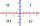In what quadrant is a -1165° angle?
• CincinnatiA map is placed on a coordinate grid. Cincinnati located at (5,4) and San Diego is located at (-10, -3). How far apart is Cincinnati from San Diego on the map? Round to the nearest tenth.
• Cone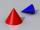If the segment of the line y = -3x +4, that lies in quadrant I, is rotated about the y-axis, a cone is formed. What is the volume of the cone?
• Coordinates of the intersection of the diagonals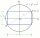In the rectangular coordinate system, a rectangle ABCD is drawn. The vertices of the rectangle are determined by these coordinates A = (2.2) B = (8.2) C = (8.6) D = (2.6) Find the coordinates of the intersection of the diagonals of the ABCD rectangle
• Comparing exponents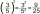A) 8 (6²)(8²) b) 16 (6²)(8²) c) (6¹+²) (8²)
• Comparing powers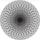How many times is number 56 larger than number 46?
• Line segmentFor the line segment whose endpoints are L[-1, 13] and M[18, 2], find the x and y value for the point located 4 over 7 the distance from L to M.
• Intersections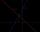Find the intersections of the function plot with coordinate axes: f (x): y = x + 3/5
• Aircraft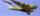The aircraft has in a fuel tank 68 hl of aviation fuel and flight consumes 3.6 liters of fuel. Identify the function, which expresses the dependence of the volume of fuel in tank on the track distance plane flew by. How many hectolites of fuel is still in
• Journey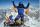The road from A to B measures 11.5 km. Firstly up the hill, then by level plane and then downhill. Tourist goes uphill at 3 km/h, on the plane 4 km/h and downhill 5 km/h. From the point, A to B went 2h 54 min back 3h 6 min. How long is the segment of the
• Symmetry by plane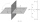Determine the coordinates of a image of point A (3, -4, -6) at a symmetry that is determined by the plane x-y-4z-13 = 0
• Slope formFind the equation of a line given the point X(8, 1) and slope -2.8. Arrange your answer in the form y = ax + b, where a, b are the constants.
• Summer tiresThree tire servants have to change the summer tires on 6 cars in 2 hours. Mark's replacement would take 4.5 hours, Jirka would do it in 3 hours and 10 minutes, and Honza in 4 hours. Will they be able to replace all tires at the desired time?
• Three points 4The line passed through three points - see table: x y -6 4 -4 3 -2 2 Write line equation in y=mx+b form